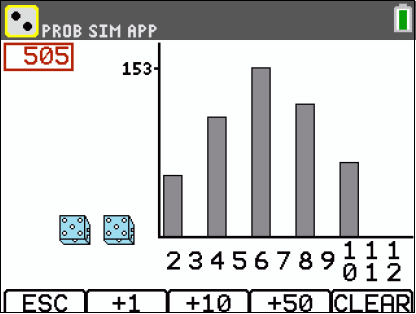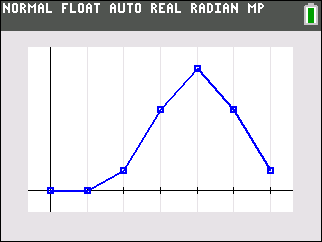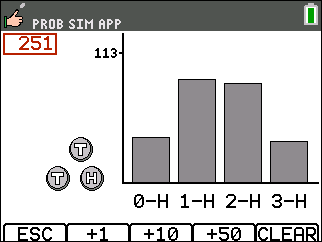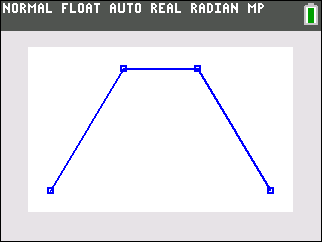••• ##### Device
• TI-84 Plus
• TI-84 Plus Silver Edition
•TI-84 Plus C Silver Edition
•TI-84 Plus CE

# Middle Grades Math: Roll the Dice

by Texas Instruments#### Overview

Students compute sample spaces for coin flips, find the various probabilities associated, and graph the probabilities using the calculator.

#### Key Steps

•Students will calculate the probability and graph the distribution of using unfair coins.

•Students will calculate the probability of all possible outcomes of tossing three coins.

•Students will graph the distribution.